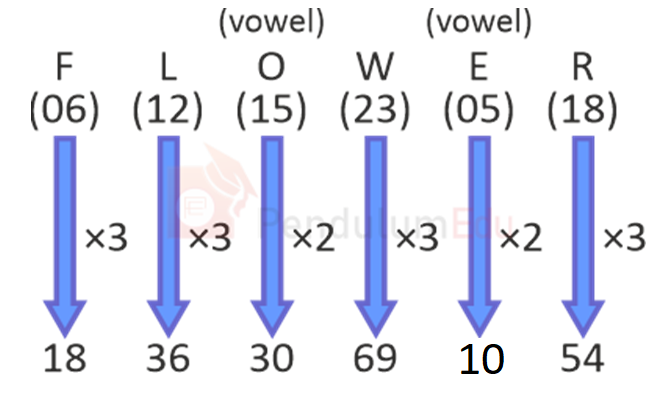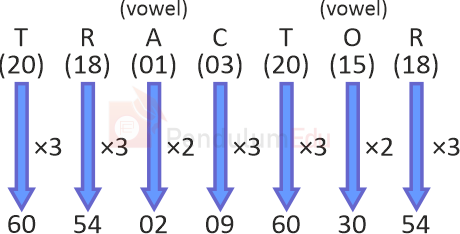Question of The Day23-12-2021

In a code language, ‘FLOWER’ is written as ‘183630693054’. How will ‘TRACTOR’ be written as in that language?

Correct Answer : a ) 60540209603054

Explanation :

According to the question

Let us assume A is numbered as 1, B as 2 and so on till Z being numbered as 26

The common logic applied here is:

Consonants are multiplied by 3 whereas vowels are multiplied by 2

Code for FLOWERSimilarly, TRACTORThus, the code for TRACTOR is 60540209603054

Hence, (a) is the correct answer.0